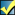A Flash Developer Resource Site

# Thread: [RESOLVED] Bubble Problems Continued

1. ##[RESOLVED] Bubble Problems Continued

So earlier I was asking for help with something else related to this that has been somewhat resolved. My problem now is that the bubbles being created by the following code are appearing in the foreground no mater where I move the layer order.

Is there any sort of code that would put the bubbles in the background? I was thinking using .swapDepths could work somehow, but I can't seem to get it to work right if it is what I should use.

PHP Code:
``` kNbrBalls = 20; gravK = -0.0;            // gravity dampK = 01;           // lower numbers make more air resistance dampCollision = .99;     // energy retained in collisions ballRadius = 15; ballWidth = ballRadius*2; SH = Stage.height; SW = Stage.width; MovieClip.prototype.handleCollisions = function() {     for (var i = mcs.length-1; i >= 1; --i) {         var x1 = mcs[i]._x;         var y1 = mcs[i]._y;         for (j = i-1; j >= 0; --j) {             var dx = mcs[j]._x - x1;             var dy = mcs[j]._y - y1;             var dist = Math.sqrt(dx*dx+dy*dy);             if (dist < ballWidth) {                 // collide - trade energy (mag1,mag2), and travel in opposite direction of collision                 var mag1 = Math.sqrt(mcs[i].vx*mcs[i].vx+mcs[i].vy*mcs[i].vy);                 var mag2 = Math.sqrt(mcs[j].vx*mcs[j].vx+mcs[j].vy*mcs[j].vy);                 mcs[j].vx = (mag1*dx/dist)*dampCollision;                 mcs[j].vy = (mag1*dy/dist)*dampCollision;                 mcs[i].vx = -(mag2*dx/dist)*dampCollision;                 mcs[i].vy = -(mag2*dy/dist)*dampCollision;             }         }     } } MovieClip.prototype.moveBall = function() {     // compute forces on ball     if (!this.isDragging) {         this.vx *= dampK;    // damping due to air resistance         this.vy *= dampK;         this.vy += gravK;    // gravity                  // bounce off floor and ceiling         if (this._y+ballRadius + this.vy >= SH ||             (this._y-ballRadius) + this.vy < 0)         {             this.vy *= -dampCollision;         }         // bounce off right and left walls         if ((this._x-ballRadius) + this.vx <= 0 ||              this._x+ballRadius + this.vx >= SW)         {             this.vx *= -dampCollision;         }         this._x += this.vx;         this._y += this.vy;     } } mcs = []; for (var i = 0; i < kNbrBalls; ++i) {   var mc = this.attachMovie("superball_mc", "mc"+i, 1+i);   mcs[i] = mc;   mc._x = ballRadius+random(Stage.width-ballWidth);   mc._y = ballRadius+random(Stage.height-ballWidth);   mc.vx = Math.random()*32-16;   mc.vy = Math.random()*32-16;   mc._width = ballRadius*2;   mc._height = ballRadius*2;   mc.onEnterFrame = mc.moveBall;   mc.stop();     choice = Math.round(Math.random()*4);     switch (choice) {     case 0 :         mc.gotoAndPlay(1);         break;     case 1 :         mc.gotoAndPlay(90);         break;     case 2 :         mc.gotoAndPlay(180);         break;     case 3 :         mc.gotoAndPlay(270);         break;     case 4 :         mc.gotoAndPlay(360);         break;         } } _root.onEnterFrame = handleCollisions;  ```Reply With Quote

2. Have you tried this: var mc = this.attachMovie("superball_mc", "mc"+i, 0-i);
¿?Reply With Quote

3. Make an empty movieclip on a lower layer, put it an instance name of "holder" and change this line of your code:

var mc = holder.attachMovie("superball_mc", "mc"+i, holder.getNextHighestDepth());Reply With Quote

4. The first method didn't work, but the second worked perfectly.Originally Posted by angelhdzMake an empty movieclip on a lower layer, put it an instance name of "holder" and change this line of your code:

var mc = holder.attachMovie("superball_mc", "mc"+i, holder.getNextHighestDepth());
Thanks angelhdzReply With Quote

as2, background, bouncing balls, bubbles, depth####Posting Permissions

• You may not post new threads
• You may not post replies
• You may not post attachments
• You may not edit your posts
•

 » Home » Movies » Tutorials » Submissions » Board » Links » Reviews » Feedback » Gallery » Fonts » The Lounge » Sound Loops » Sound FX » About FK » Sitemap